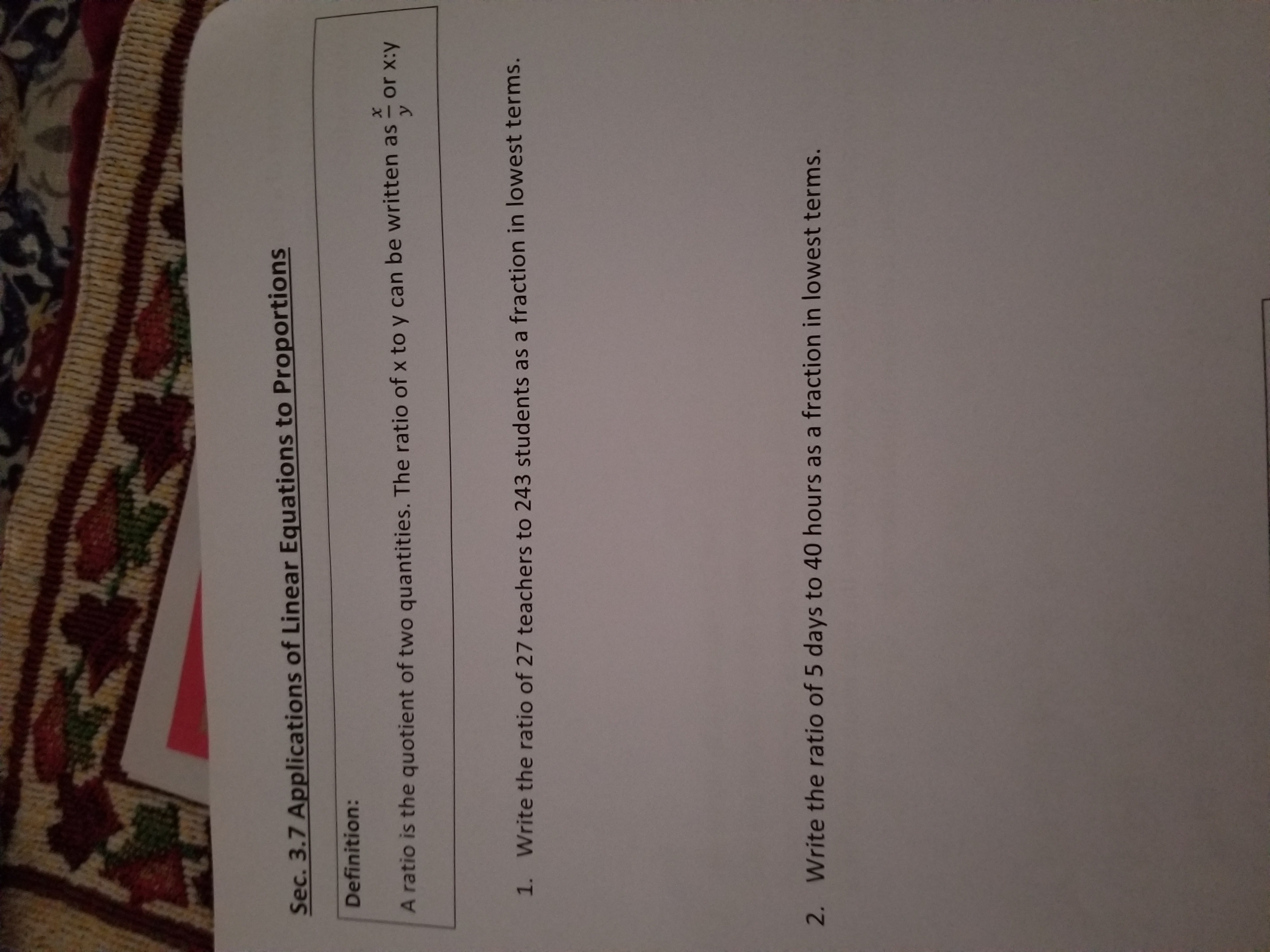# Sec. 3.7 Applications of Linear Equations to Proportions Definition: х or x:y A ratio is the quotient of two quantities. The ratio of x to y can be written as 1. Write the ratio of 27 teachers to 243 students as a fraction in lowest terms. 2. Write the ratio of 5 days to 40 hours as a fraction in lowest terms.

Questionhelp_outlineImage TranscriptioncloseSec. 3.7 Applications of Linear Equations to Proportions Definition: х or x:y A ratio is the quotient of two quantities. The ratio of x to y can be written as 1. Write the ratio of 27 teachers to 243 students as a fraction in lowest terms. 2. Write the ratio of 5 days to 40 hours as a fraction in lowest terms. fullscreen